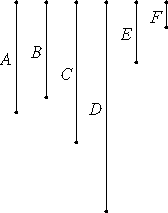# Proposition 24

If two numbers are relatively prime to any number, then their product is also relatively prime to the same.

Let the two numbers A and B [each] be relatively prime to a number C, and let A multiplied by B make D.

I say that C and D are relatively prime.If C and D are not relatively prime, then some number E measures C and D.

VII.23

Now, since C and A are relatively prime, and a certain number E measures C, therefore A and E are relatively prime.

VII.16

Let there be as many units in F as the times that E measures D. Then F also measures D according to the units in E.

VII.Def.15

Therefore E multiplied by F makes D. Also, A multiplied by B makes D, therefore the product of E and F equals the product of A and B.

VII.19

But, if the product of the extremes equal that of the means, then the four numbers are proportional. Therefore E is to A as B is to F.

But A and E are relatively prime, numbers which are relatively prime are also the least of those which have the same ratio, and the least numbers of those which have the same ratio with them measure those which have the same ratio the same number of times, the greater the greater, and the less the less, that is, the antecedent the antecedent and the consequent the consequent, therefore E measures B.

But it also measures C, therefore E measures B and C which are relatively prime, which is impossible.

VII.Def.12

Therefore no number measures the numbers C and D. Therefore C and D are relatively prime.

Therefore, if two numbers are relatively prime to any number, then their product is also relatively prime to the same.

Q.E.D.

## Guide

This proposition says that if both a and b are relatively prime to c, then ab is also relatively prime to c.

#### Outline of the proof

Assume that two numbers a and b are each relatively prime to a third number c.

Suppose their product ab is not relatively prime to c. Then there is some number e (greater than 1) that divides both ab and c. Now, since e divides c, and c is relatively prime to a, therefore, by VII.23, e is also relatively prime to a.

Let f be the number ab/e. Then e : a = b : f. Since e and a are relatively prime, then, by VII.21, e : a is in lowest terms. Therefore, by VII.20, e divides b. But then e divides both b and c contradicting the assumption that b and c are relatively prime.

Therefore, the product ab is also relatively prime to c.

#### Use of this proposition

This proposition is used in the next two and in IX.15.